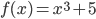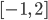### Day 35 - Increasing/Decreasing Functions - 02.23.16

 Update Summative Exam 1Average = 77%Calculus Equations/Theorems What are critical numbers?numbers needed to solve problemsvalues of x that cause a derivative to be equal to zerovalues of y that cause a derivative to be equal to zerovalues of x that cause a derivative to be undefinedboth b and dHow are relative extrema related to critical numbers?There is no relationship between critical numbers and relative extrema.Every relative extrema occurs at a critical number.Every critical number has a relative extrema.Critical numbers are only found at relative extrema.none of the aboveFind the critical number(s) of the following:x = 1x = -1x = 0undefinednone of the aboveFind the relative extrema for the following function:(1, 6)(-1, 4)(0, 5)no relative extremanone of the aboveFind and describe all extrema for the following function:on the intervalAbsolute max: (-1, 4) | Absolute min: (2, 13) | No relative extremaAbsolute min: (-1, 4) | Absolute max: (2, 13) | Relative min: (0, 5)Absolute min: (-1, 4) | Absolute max: (2, 13) | No relative extremaNo extremanone of the aboveReviewPre-calculusExtremaMinimaMaximaAbsolute and Relative Extrema (video)Interval NotationExtrema (video)/Critical Numbers ((checkpoints)How can extrema be defined for a function?How can critical numbers be calculated using derivatives?How are critical numbers related to extrema?How does Extreme Value Theorem work?LessonChallenge 3.2Using only calculus, how can we find extrema?Sketch a function with extrema.See me for hints.Increasing/Decreasing Functions (video)Checkpoints Exit Ticket Posted on the board at the end of the block.HomeworkStudy! Help Request List Standard(s) APC.7Analyze the derivative of a function as a function in itself.Includes:comparing corresponding characteristics of the graphs of f, f', and f''​defining the relationship between the increasing and decreasing behavior of f and the sign of f'​translating verbal descriptions into equations involving derivatives and vice versaanalyzing the geometric consequences of the Mean Value Theorem;defining the relationship between the concavity of f and the sign of f"; and ​identifying points of inflection as places where concavity changes and finding points of inflection.APC.8Apply the derivative to solve problems.Includes:​analysis of curves and the ideas of concavity and monotonicityoptimization involving global and local extrema;modeling of rates of change and related rates;use of implicit differentiation to find the derivative of an inverse function;interpretation of the derivative as a rate of change in applied contexts, including velocity, speed, and acceleration; anddifferentiation of nonlogarithmic functions, using the technique of logarithmic differentiation.**AP Calculus BC will also apply the derivative to solve problems.Includes:​analysis of planar curves given in parametric form, polar form, and vector form, including velocity and acceleration vectors;​numerical solution of differential equations, using Euler’s method;​l’Hopital’s Rule to test the convergence of improper integrals and series; and​geometric interpretation of differential equations via slope fields and the relationship between slope fields and the solution curves for the differential equations.